# Constructing a sphere with 455 cups

Paper cups do not follow a hexagonal pattern and the cones do not meet at a single point, but they do make a gigantic sphere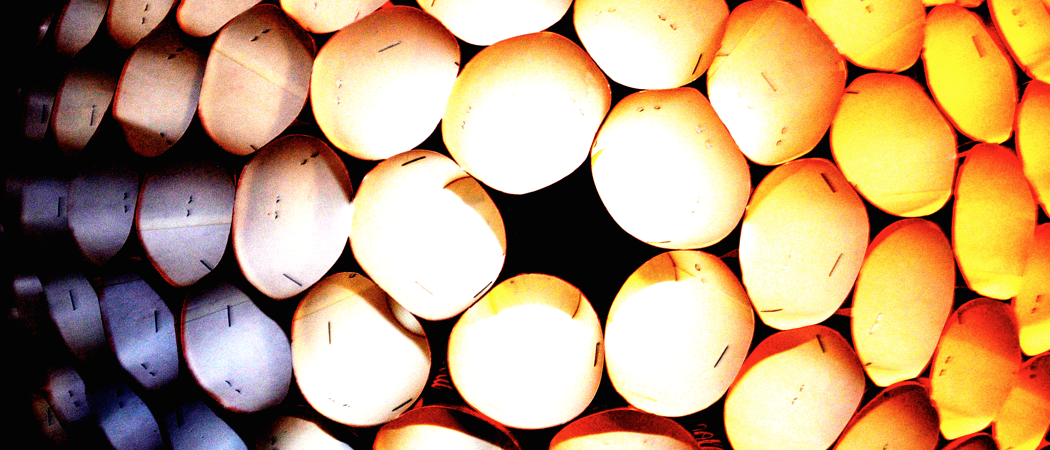A few weeks ago we set this problem: if a cup is a section of a cone and we put one right next to another, and then add a third, and a fourth and so on, we end up with a gigantic sphere. The problem is easy: how many cups do we need to make a sphere? Well, now we have the answer!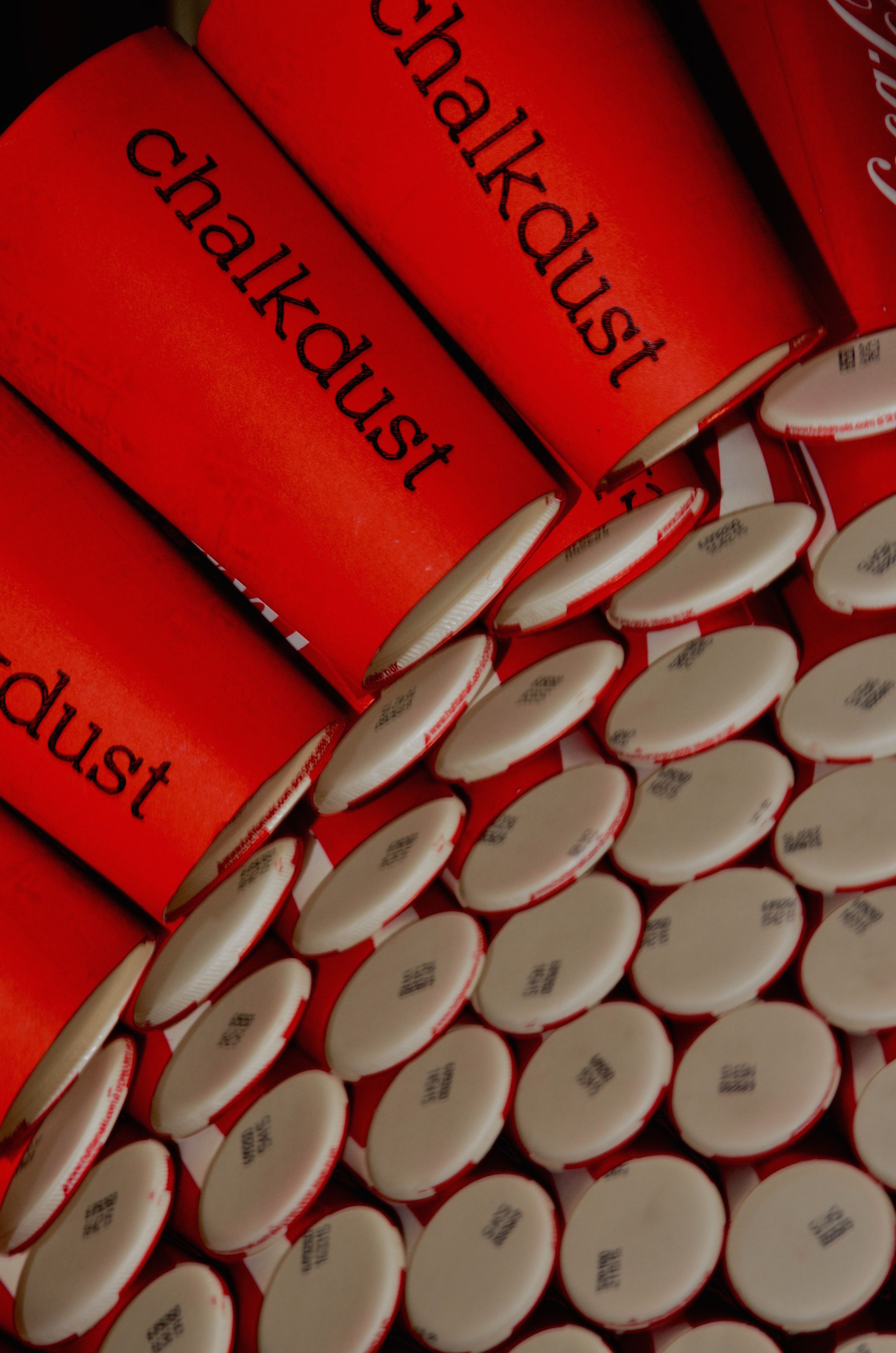We decided to make it a contest, and so for a period of four weeks, our readers could submit their own answer, based on their maths and intuition.

We constructed the sphere and we used 455 paper cups to finish it. The sphere is gigantic and exactly one person guessed the right number! Well done Katy!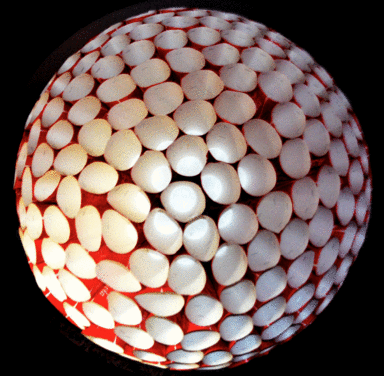We received 85 valid answers (and a big thank you to all of our readers who took the time to submit an answer or tried the problem) and most of them included hints as to what they did to estimate their number of cups. Here are some of the answers we liked the most:Worked out the cone angle, extrapolated how many cups you could then connect to create a great circle, and then used the standard circle packing model (one fewer on each row) to guess the number of cups.

—S. W.The inner radius of the sphere (at the bottom of the cups) is ~23.4 cm. Tight-packing cups in a (flat) hexagonal pattern has a density of ~0.9069 (thank you, Lagrange). So approximating the sphere as flat, which doesn’t immediately seem silly, and densely packing the circles on its surface, I get that the number of cups is 79.5. Then I’ll give you some leeway and guess for a nice round 80.

— J. T.Every cup after the second introduces another hole. I calculated the area of the cup and the hole and then divided. Should probably bodge it a bit for a non-perfect stacking, but I’m a physicist, not an engineer.

—H. B.Packing circles hexagonally can’t work all the way round because of the Euler characteristic (it works fine for a torus). Therefore, I modelled this as an icosahedron (so hexagonal packing over the majority of the face, with discrepancies at the vertices). I found the radius of the sphere (2D problem), calculated the area of the sphere, found 1/20 of this, and modelled it as an equilateral triangle. I found that you could fit slightly less than 5 cups on the length of a side, which means slightly less than 15 cups per face of the icosahedron. This means that the answer should be slightly less than 300, so I have guessed 285. 🙂

—M. H.…By symmetry arguments, you would probably like the number of cups to be divisible by 12 and possibly 60.  If you think about the face of each cup as a polygon (actually a hexagon or pentagon), then 300 corresponds nicely to the $G(1,5)$ Goldberg polyhedron.

—D. R.Way too complicated maths.

—D. C.Assuming there are no gaps between the cups’ top or bottom surfaces, and that they stay rigid and don’t deform under their own weight, it can be solved a few different ways: by summing together the cups’ top areas, bottom areas, or volumes. I made a graphic to summarise all this on my website.

—A.

## Wisdom of the crowd

We received 85 valid answers. The mean response was 502 cups (since a couple of answers were very large), but the median response was much lower at 333 cups. Although 85 people is not a huge crowd, the wisdom of the crowd is fairly accurate: 85 people trying to solve the problem, each one using different assumptions and techniques, give a reasonably good answer overall.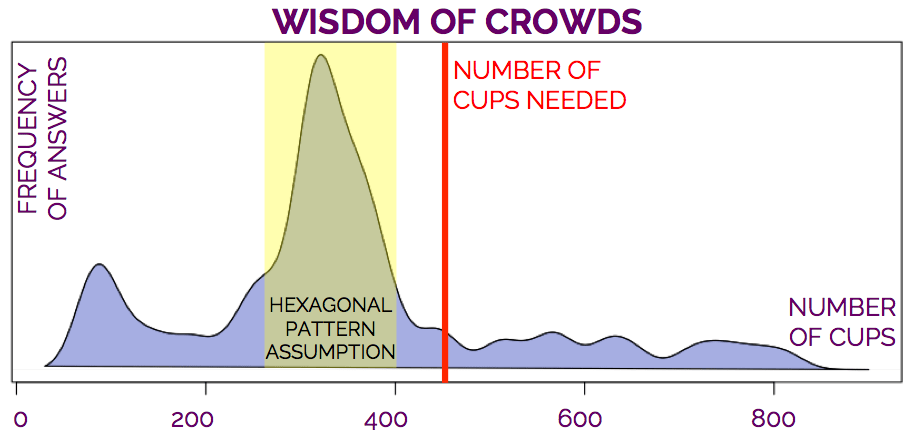Interestingly, the most frequent answer was exactly 318 cups (and most of them are around that number), which is the result of assuming a hexagonal pattern and that the cones meet at a single point at the centre of a perfect sphere. Perhaps we experienced the wisdom of the mathematicians and that explains the bias in the 85 answers!

## Hexagonal pattern or not?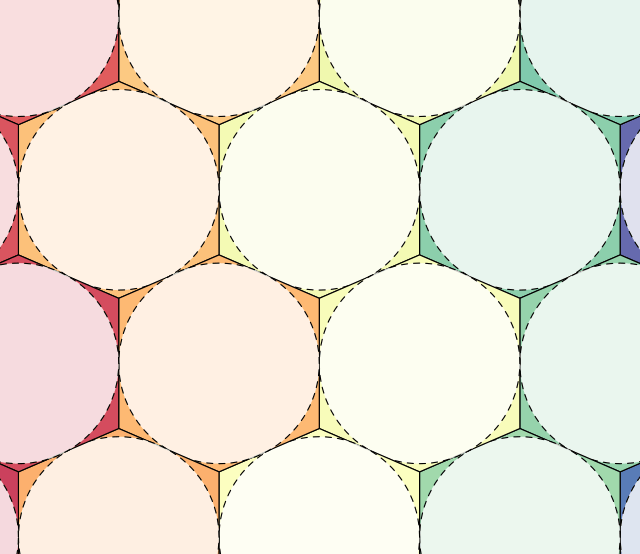Do the cups (either their lower or upper part) actually form a hexagonal pattern? No.

Whilst we were constructing the sphere we discovered that we could force the upper part of the paper cups into a hexagonal pattern, but by forcing them, we were constructing a gigantic flat surface.

Eventually, as we were constructing the sphere, we encountered gaps which could not be naturally filled with a cup, and so we leave them as gaps, without forcing and without following a hexagonal pattern.## Do the vertices of the cones meet in a single point?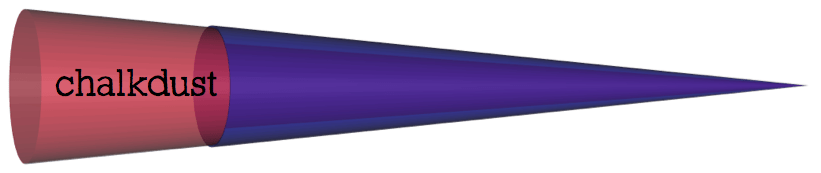The height of a cone is 35.1cm, and so by measuring the sphere, we can test whether the vertices of the cones actually meet at a single point, and the answer, surprisingly, is no. They don’t. The radius of the sphere is 42cm, meaning that, if we had built the sphere with full cones (and not just sections of it), then we would have an empty space in the centre (with the shape of a sphere with a radius of 7cm).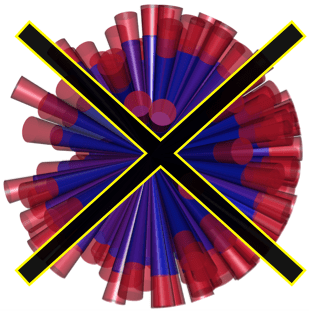## Perfect sphere?

The paper cups are not rigid and we are not professional sphere builders, so there were some deformations along the way, which of course change the result a bit. In particular, the cups were slightly squashed, changing the angle of the cone. Perhaps we would obtain a better sphere with more rigid cups and engineering experience!